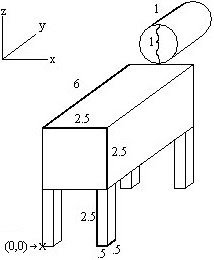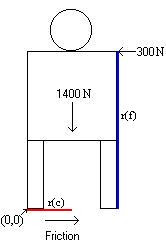Thursday, December 18, 2008

## Physics of Cow TippingOn a late night with nothing to do but hang around cow pastures, the idea of cow-tipping may easily cross your mind. Assuming there is a cow asleep and standing up, you next want to figure out how much force is required – if applied perpendicularly – to tip the cow over.

(image on right is from shirtstats.com)

The Statics of Cow Tipping has provided approximate values and has performed calculations. Here, we will discuss the process undertaken as well as a critique of the work.

First, let us approximate the following dimensions of a cow:

Body (rectangular prism) = lwh = 2.5*2.5*6 = 37.5 ft3

Head (cylinder) = pi*r2*h = 3.14*.52*1 = .785 ft3

Legs x4 (rectangular prism) = lwh = .5*.5*2.5 = .625 ft3

From here, we can find the center of mass for each body part. Shown below is the table from The Statics of Cow Tipping (source).

## Center of Mass

Part Volume XCenter YCenter ZCenter X(V) Y(V) Z(V)
Body 37.5 1.25 3 3.75 46.875 112.5 140.625
Head .785 1.25 6.5 5.5 .98125 5.1025 4.3175
Leg 1 .625 .25 .25 1.25 .15625 .15625 .78125
Leg 2 .625 2.25 .25 1.25 1.40625 .15625 .78125
Leg 3 .625 .25 2.25 1.25 .15625 1.40625 .78125
Leg 4 .625 2.25 2.25 1.25 1.40625 1.40625 .78125
Total 40.785 ---- ---- ---- 50.98125 120.7275 148.0675In addition to leaving out units (which I have added in), the site fails to explain where exactly the body parts are located and to what point are the Center values relative. To the left is my own rendition of the cow, which is drawn more closely to scale and includes the point of relativity, noted as the origin point (0,0).

Continuing on in the table, the columns labeled X(V), Y(V), and Z(V) are found by multiplying each part’s volume by its respective XCenter, YCenter, and ZCenter distances (columns 3-5). Of course, this is assuming that the density of the cow is uniform. In reality, the density is not uniform, thereby changing where the center of gravity is located for each part. However, we will ignore reality for this analysis. The volume components of each part are totaled and then divided by 40.785ft3, the total volume of the cow. These answers signify what distance the overall center of gravity is from the point of relativity. The distances are as follows:

XCenter = 1.25 ft

YCenter = 2.96 ft

ZCenter = 3.63 ft

Now we move on to equilibrium/torque calculations. We will assume the same given values as The Statics of Cow Tipping:

mass of cow = 1400 lbs

height of horizontal pushing force = 5 ft

horizontal pushing force = 300 lbs

force of friction = 1400*.45 = 630 lbs

(the cow won't slide)

Although we’ll use the same equation, torque = force*radius, our radius will assume that the cow’s left hind and fore leg remain on the ground. Shown below are two images. The force vector schematic on the left is editted from The Statics of Cow Tipping (source) and includes the forces due to friction, pushing, and gravity on the cow. For the image on the right, the radii are labeled as follows:To find the correct radius, we need to consider the moment arm of the force. Since the moment arm is the perpendicular distance from the axis of rotation to the extended line of force, we see that r(f) is indeed perpendicular to the pushing force and r(c) is indeed perpendicular to the force of gravity on the cow.

We can easily find that r(f) = 5 ft and r(c) = 1.25 ft. Now the difference in torque is 1400*1.25-300*5=250 lbs/ft. We need the torque to be less than zero for the pushing force to be sufficient enough to overcome the cow's turning force, so it is clear that one person is not enough to tip a cow.

We're determined to tip the cow, so let's add more people. Assuming the people have identical pushing height and force and will stand in a way so as to push the cow in a uniform manner, then we can calculate that the minimum number of people required to tip a cow is 1400*1.25 < n="2.

Our results agree with The Statics of Cow Tipping. However, we must also consider some real life truths. Most likely, a real cow's legs will move or tilt when the cow is pushed. On the plus side, this moves the center of gravity, making it easier to tip the cow as the shift will shorten r(c) with time while r(f) remains the same. Unfortunately, the cow would sure counter-balance, making it more difficult to tip. Even so, these calculations are still good approximations and as such, leave the myth of cow tipping open to testing!(above image is from freewebs.com)

References:

Semke, Matt. "The Statics of Cow Tipping", http://emweb.unl.edu/Mechanics-Pages/Matt-Semke/The%20Statics%20of%20Cow%20Tipping.htm

Images:

Author unknown. "Google Image Search on cow tipping", http://s3-external-1.amazonaws.com/wootdesigncontestentries/Wobblytoddles/Cow_tipping-hihv9v-d.jpg

Semke, Matt. "The Statics of Cow Tipping", http://emweb.unl.edu/Mechanics-Pages/Matt-Semke/The%20Statics%20of%20Cow%20Tipping.htm

Author unknown. "Google Image Search on cow tipping", http://www.freewebs.com/katseyeview/sleeping%20cow.jpg